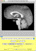# Calculating Structure Volumes

### MultiTracer version 2

Once structures have been traced, their volumes can be calculated. For the volume of a structure to be defined, it must be traced on more than one plane and the planes on which it is traced must all be contiguous.

Volumes can be calculated by doing the following:

1. Choose "Analyze Contours" from the "Analyze" menu in the main window
2. In the displayed table, the volumes of each valid structure are shown:
Classic Vol
This is the volume calculated by summing the areas for each plane and multiplying by the slice thickness
Trap Vol
This is the volume calculated by assuming that the structure extends from the center of the first plane on which it was drawn to the center of the last plane on which it was drawn with areas varying linearly when moving from the center of one plane to the center of the next
Frust Vol
This is the volume calculated by assuming that the structure extends from the center of the first plane on which it was drawn to the center of the last plane on which it was drawn with the square root of areas varying linearly when moving from the center of one plane to the center of the next
X Min
This is the lowest x-coordinate found for the structure as measured in calibrated real world units with the origin located at the center of the first voxel in the original image
Y Min
This is the lowest y-coordinate found for the structure as measured in calibrated real world units with the origin located at the center of the first voxel in the original image
Z Min
This is the lowest z-coordinate found for the structure as measured in calibrated real world units with the origin located at the center of the first voxel in the original image
X Max
This is the highest x-coordinate found for the structure as measured in calibrated real world units with the origin located at the center of the first voxel in the original image
Y Max
This is the highest y-coordinate found for the structure as measured in calibrated real world units with the origin located at the center of the first voxel in the original image
Z Max
This is the highest z-coordinate found for the structure as measured in calibrated real world units with the origin located at the center of the first voxel in the original image
3. If volume values in the table are zero for a particular structure, you can choose that structure in the control panel in the main window and then select "Check Structure Integrity" under the "Analyze" menu in the main window. A message dialog will either confirm that the structure has been correctly drawn or will identify the underlying problem
4. You can choose "Copy" from the "Edit" menu of the table's window to copy all of the data in tab delimited format that can be pasted into a spreadsheet©2001-2012 Roger P. Woods, M.D.

Modified: August 26, 2012